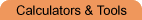Home    |    Teacher    |    Parents    |    Glossary    |    About UsEmail this page to a friendSelect Tool· Calculators · Converters · Equation Solvers · GraphersResources· Cool Tools · Formulas & Tables · References · Test Preparation · Study Tips · Wonders of MathSearchGraphing and Plotting Free! Instant graphs from your computer! Graph equations and inequalities Full-featured instant graphing solution, no Java needed. You can print graphs too! Features: Generate a plot of almost any function or relation found in high school and undergraduate college mathematics. It will plot functions given in the form y = f(x), such as y = x2 or y = 3x + 1, as well as relations of the form f(x,y) = g(x,y), such as x2 + y2 = 4. The advanced plot allows you to graph multiple equations on the same graph. Applet function plotter You type in an equation and this applet will draw your graph on an x,y coordinate grid. You can graph one, two, or more equations and compare graphs. (From maths online, University of Vienna) Note: When you click on this link, a new window will open. It may take a few minutes to load - please be patient! Equations are entered in terms of x, such as: 2*x+5 (the asterisk must always be used for multiplication). More help with typing in equations in available once the applet is running.